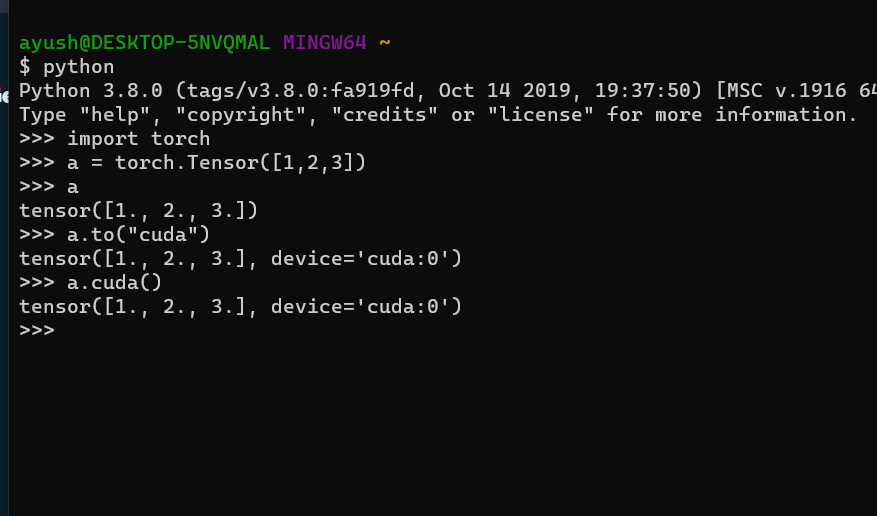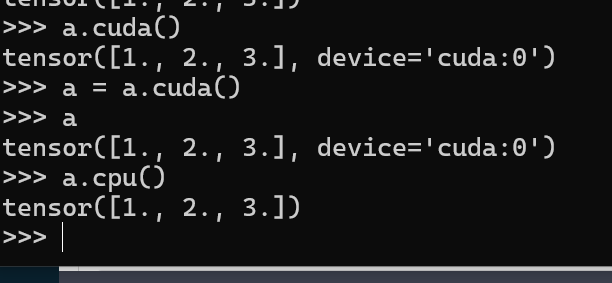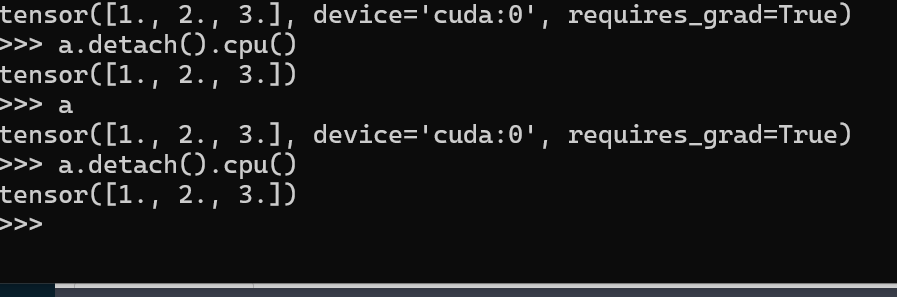Open in App
Not now

# How to Move a Torch Tensor from CPU to GPU and Vice Versa in Python?

• Last Updated : 25 May, 2022

In this article, we will see how to move a tensor from CPU to GPU and from GPU to CPU in Python.

## Why do we need to move the tensor?

This is done for the following reasons:

• When Training big neural networks, we need to use our GPU for faster training. So PyTorch expects the data to be transferred from CPU to GPU. Initially, all data are in the CPU.
• After doing all the Training related processes, the output tensor is also produced in the GPU. Often, the outputs from our Neural Networks need preprocessing. Most preprocessing Libraries don’t have support for Tensors and expect a NumPy array. NumPy does not store data in GPU so it expects Data to be in CPU.

Now that we know why do we need to do these operations, let’s see how can we do it in PyTorch tensor.

### CPU to GPU

To move our Tensors from CPU to GPU we use either one of these commands:

`Tensor.cuda() `
`Tensor.to("cuda")`

Example:### GPU to CPU

Now for moving our Tensors from GPU to CPU, there are two conditions:

1. Tensor with required_grad = False, or
2. Tensor with required_grad = True

Example 1: If required_grad = False, then you can simply do it as:

`Tensor.cpu()`Example 2: If required_grad = True, then you need to use:

`Tensor.detach().cpu()`My Personal Notes arrow_drop_up
Related Articles